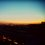# I am thinking a bit stupidly but here comes a thought:

Let's find the indefinite integral of $\dfrac{e^x}{x}.$

....I know it's $\text{Ei}(x)+C$ but let's do it anyways

So firstly, I'm gonna let $\dfrac{1}{x}=t,$ leading us to $x=\dfrac{1}{t}.$

Differentiating gives $dx=-\dfrac{1}{t^2}dt,$ and therefore:

\begin{aligned} \int \frac{e^x}{x}dx &= \int \frac{1}{x}\cdot e^x dx \\ & =\int t \cdot e^{\frac{1}{t}}\cdot\left(-\dfrac{1}{t^2}\right) dt \\ & =- \int \frac{e^{\frac{1}{t}}}{t} dt \\ & =- \int \frac{e^{\frac{1}{x}}}{x} dx \\ \end{aligned}

From above, we get:

$\displaystyle \int \frac{e^x}{x} dx + \int \frac{e^{\frac{1}{x}}}{x} dx = 0 + (C)$

$\displaystyle \int \frac{e^x+e^{\frac{1}{x}}}{x} dx = 0 + (C)$

Differentiating both sides gives:

$\displaystyle \frac{e^x + e^{\frac{1}{x}}}{x} = 0$

....then...

$\displaystyle e^x+e^{\frac{1}{x}}=0$...?!

I didn't expect this to happen but I have this terrible migraine to think more deeply about this ._.

Yes I am stupid, sorry ;;>_>Note by Boi (보이)
3 years, 9 months ago

This discussion board is a place to discuss our Daily Challenges and the math and science related to those challenges. Explanations are more than just a solution — they should explain the steps and thinking strategies that you used to obtain the solution. Comments should further the discussion of math and science.

When posting on Brilliant:

• Use the emojis to react to an explanation, whether you're congratulating a job well done , or just really confused .
• Ask specific questions about the challenge or the steps in somebody's explanation. Well-posed questions can add a lot to the discussion, but posting "I don't understand!" doesn't help anyone.
• Try to contribute something new to the discussion, whether it is an extension, generalization or other idea related to the challenge.
• Stay on topic — we're all here to learn more about math and science, not to hear about your favorite get-rich-quick scheme or current world events.

MarkdownAppears as
*italics* or _italics_ italics
**bold** or __bold__ bold
- bulleted- list
• bulleted
• list
1. numbered2. list
1. numbered
2. list
Note: you must add a full line of space before and after lists for them to show up correctly
paragraph 1paragraph 2

paragraph 1

paragraph 2

[example link](https://brilliant.org)example link
> This is a quote
This is a quote
    # I indented these lines
# 4 spaces, and now they show
# up as a code block.

print "hello world"
# I indented these lines
# 4 spaces, and now they show
# up as a code block.

print "hello world"
MathAppears as
Remember to wrap math in $$ ... $$ or $ ... $ to ensure proper formatting.
2 \times 3 $2 \times 3$
2^{34} $2^{34}$
a_{i-1} $a_{i-1}$
\frac{2}{3} $\frac{2}{3}$
\sqrt{2} $\sqrt{2}$
\sum_{i=1}^3 $\sum_{i=1}^3$
\sin \theta $\sin \theta$
\boxed{123} $\boxed{123}$

## Comments

Sort by:

Top Newest

You forgot about the arbitrary constant of integration.

- 3 years, 9 months ago

Log in to reply

Hmm...

then let $\displaystyle \int \frac{e^x}{x}dx+\int \frac{e^{\frac{1}{x}}}{x}dx=C,$ but when you differentiate both sides,

$\dfrac{e^x+e^{\frac{1}{x}}}{x}=0.$

- 3 years, 9 months ago

Log in to reply

[ this comment is wrong ]

- 3 years, 9 months ago

Log in to reply

Yes

The integral of 1 - cos^2 x is

The integral of 1 - the integral of cos^2 x

= x - the integral of cos^2 x + C

Therefore

The integral of sin^2 x + the integral of cos^2 x = x + C

Differentiate and we get

sin^2 x + cos^2 x = 1

- 3 years, 9 months ago

Log in to reply

Awhh damn it. I'll try to fix up my reply and answer your question shortly....

- 3 years, 9 months ago

Log in to reply

Arghhh, curses! I can't properly explain it. I think got something to do with inverse functions and 1-1 or something.

Summoning the great @Mark Hennings

- 3 years, 9 months ago

Log in to reply

Suppose that we write $F(x) \; = \; \int_1^x \frac{e^u}{u}\,du \hspace{2cm} G(x) \; = \; \int_1^x \frac{e^{\frac{1}{u}}}{u}\,du$ Then the substitution $v = u^{-1}$ gives $F(x) \; = \; -\int_1^{x^{-1}} \frac{e^{\frac{1}{v}}}{v}\,dv \; = \; -G(x^{-1})$ and hence $F(x) + G(x^{-1}) \; = \; 0$ What you are saying is that $F(x) + G(x) \; = \; c$ which is not true. You are making the substitution $t = x^{-1}$, and then replacing $t$ by $x$, instead of by $x^{-1}$. Since you are working with indefinite integrals, you cannot exchange the variables freely if they already have an interrelationship.

- 3 years, 9 months ago

Log in to reply

AHHH AH AH AH OKE THANKS now I understand, really, thank you!

- 3 years, 9 months ago

Log in to reply

$\int \frac{e^{1/t}}{t} dt \neq \int \frac{e^{1/x}}{x} dx$. Remember $x=\frac{1}{t}$, and we are talking about indefinite integrals.

- 3 years, 8 months ago

Log in to reply

Yeah... Someone told me that in the below chain of comments and...

....I feel even dumber now. x'D

- 3 years, 8 months ago

Log in to reply

×

Problem Loading...

Note Loading...

Set Loading...